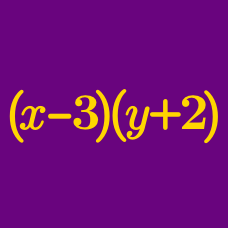Algebra

# Solving Identity Equations

How many real values of $x$ satisfy the following equation $9^x - 6 \cdot 3^x - 55 = 0$?

If the equation $3x+4y+5=ax+by+c=-dx-ey-f$ holds for all numbers $x$ and $y$, what is the value of $cf-be-ad$?

Given that $ax+4y+cz+23=7x+by+4z+d$ is an algebraic identity in $x$, $y$ and $z$, what is the value of $a+b+c+d$?

Given that $ax^2+bx+c+7x^2+8x-12=0$ is an algebraic identity, what is the value of $abc$?

If the following holds for all real numbers $x$ and $y$, $(ax+by+c)+(5x+5y-19)=0,$ what is the value of $a+b+c$?

×